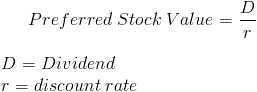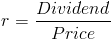# Preferred Stock

PV of Preferred Stock Calculator (Click Here or Scroll Down)The formula shown is for a simple straight preferred stock that does not have additional features, such as those found in convertible, retractable, and callable preferred stocks.

A preferred stock is a type of stock that provides dividends prior to any dividend paid to common stocks. Apart from having preference for dividend payouts, preferred stocks generally will have preference of asset allocation upon insolvency of the company, compared to common stocks. Because of these preferences, preferred stock is generally considered to be more secure than common stock and similar to a debt financial instrument, i.e., a bond. Despite the similarities, bonds do have preference for the same reasons and are generally considered more secure, ceteris paribus.

The formula for the present value of a preferred stock uses the perpetuity formula. A perpetuity is a type of annuity that pays periodic payments infinitely. As previously stated, preferred stocks in most circumstances receive their dividends prior to any dividends paid to common stocks and the dividends tend to be fixed. With this, its value can be calculated using the perpetuity formula.

## Example of Preferred Stock Value Formula

An individual is considering investing in straight preferred stock that pays \$20 per year in dividends. It has been determined that based on risk, the discount rate would be 5%. The price the individual would want to pay for this security would be \$20 divided by .05(5%) which is calculated to be \$400.

## Alternative Formula

The formula could be reworked to find the rate or return by dividing the fixed dividend payout by the price.For example, if the price is \$40 per share and the annual dividend is \$4, the rate would be .10 or 10%.

New to Finance?